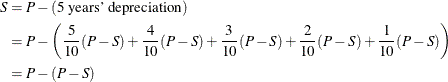Details

Sum-of-Years Digits

An asset often loses more of its value early in its lifetime. A method that exhibits this dynamic is desirable.

Assume an asset depreciates from price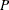to salvage value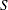in
years. First compute the sum-of-years as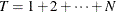. The depreciation for the years after the asset’s purchase is:

Table 59.1: Sum-of-Years General Example

Year Number

Annual Depreciation

first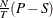second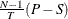third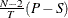final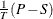For the ith year of the asset’s use, the annual depreciation is: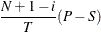For our example,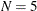and the sum of years is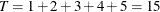. The depreciation during the first year is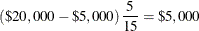Table 59.2 describes how Declining Balance would depreciate the asset.

Table 59.2: Sum-of-Years Example

Year

Depreciation

Year-End Value

1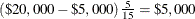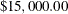2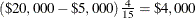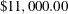3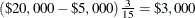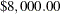4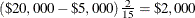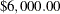5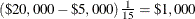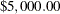As expected, the value afteryears is.